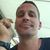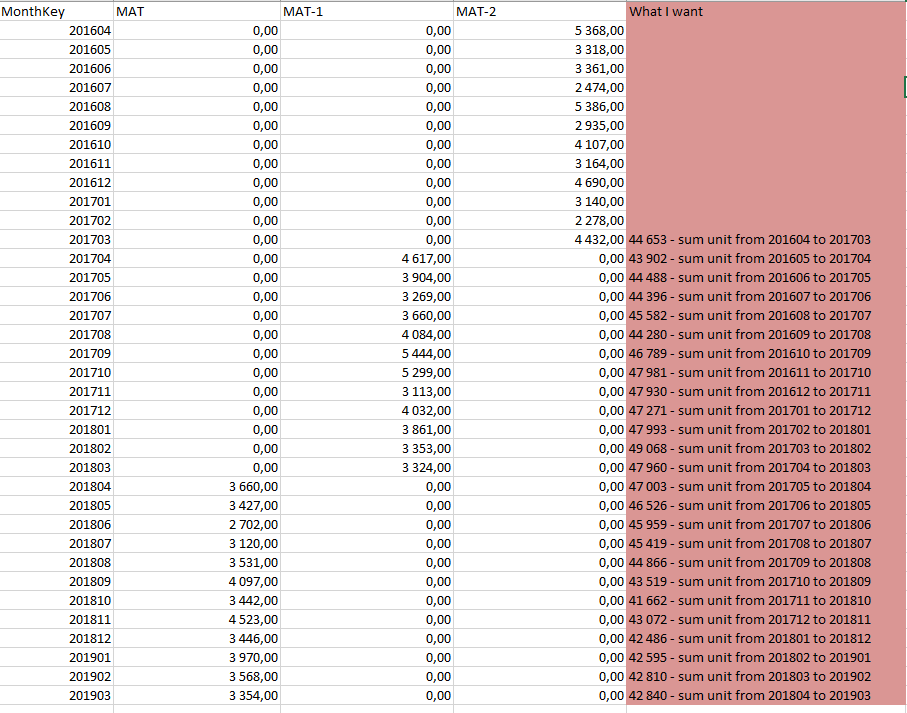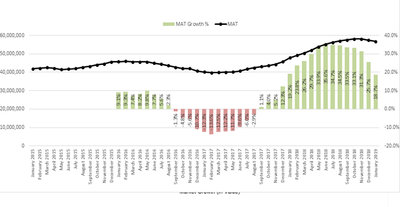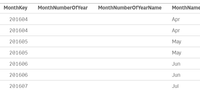# New to Qlik Sense

If you’re new to Qlik Sense, start with this Discussion Board and get up-to-speed quickly.

cancel
Showing results for
Did you mean:Partner

## MAT (Moving Annual Total ) calculation for a specific month

Hi all

I have a tricky problem.

I will create a line diagram that will show the sum of unit in MAT with year-month dimension for last 3 years.
MAT should displayed by a line, where each data point is MAT calculation for a specific month. For instance, August 18 will show (MAT August 18 = sum of measure from Sept 17 to Aug 18)

I have create measures for  MAT  CY,  MAT-1 LY  and MAT-2 2 Years back

MAT:  Sum({=\$(=Max(MonthKey)-99)”} >} Units)

MAT1:  Sum({=\$(=Max(MonthKey)-199)  < \$(=Max(MonthKey)-99) ”} >} Units)

MAT2: Sum({=\$(=Max(MonthKey)-299)  < \$(=Max(MonthKey)-199) ”} >} Units)

I tried this calculation I got from this forum

Dimension: MonthKey

FirstSortedValue({<MonthKey = {\$(=Max({<Year = {\$(=Max(Year))}>}MonthKey))}, Year = {"\$(='<=' & Max(Year))"}, Month>}Aggr(RangeSum(Above(Sum({<MonthKey>}Units), 0, 36)), MonthKey),
-Aggr(Only({<MonthKey>}MonthKey), MonthKey))

But it just give me the total

I'm not aloud to change anything in the script!

This is want I want (Se red):I hope someone can help me!

2 RepliesMVP

You want to one year back calc. for MAT-3? If so, May be use this?

FirstSortedValue({<MonthKey = {">=\$(=AddMonths(Max({<Year = {\$(=Max(Year))}>}MonthKey),-12))<=\$(=Max({<Year = {\$(=Max(Year))}>}MonthKey))"}, Year = {"\$(='<=' & Max(Year))"}, Month>} Aggr(RangeSum(Above(Sum({<MonthKey>}Units), 0, 36)), MonthKey), -Aggr(Only({<MonthKey>}MonthKey), MonthKey))

Else, Can I get that logic behind?

Before develop something, think If placed (The Right information | To the right people | At the Right time | In the Right place | With the Right context)Partner
Author

Hi Anil and thanks:)

I think I fix the first part:)

This is what I want to do:

The graph below contains both MAT growth % and MAT actual (calculated for each month of the year). Growth is displayed by bars. Each bar should display Growth MAT calculated for a specific month. For instance, a bar for August 18 will show (MAT August 18- MAT August 17)/ MAT August 17.I'm done with the black line , the MAT Actual with this expression: rangesum(above(total sum(Units),0,12))

Now I would like help with MAT Growth%. As the green bars show. I already have expressions for MAT, MAT1 and MAT2 for another purpose. They look like this:

MAT:

Sum({<MonthKey  =  {“>=\$(=Max(MonthKey) - 99)”}  >}  Units )

/

Round(Sum( {<MonthKey  =  {“>=\$(=Max(MonthKey) - 199)  < \$(=Max(MonthKey) - 99) ”} >} Units), 0.0001) – 1

MAT1:

Sum({<MonthKey  =  {“>=\$(=Max(MonthKey) - 199) < \$(= Max(MonthKey) – 99 ”}  >}  Units)

/

Round(Sum( {<MonthKey  =  {“>=\$(=Max(MonthKey) - 299)  < \$(=Max(MonthKey) - 199) ”} >} Units ), 0.0001) – 1

MAT2:

Sum({<MonthKey  =  {“>=\$(=Max(MonthKey) - 199) < \$(= Max(MonthKey) – 99 ”}  >}  Units )

/

Round(Sum( {<  >} Units), 0.0001) – 1)))

MonthKey =Maybe I can use the same calc. as for MAT actual, but put the Growth expression in the sum - rangesum(above(total sum(Here,0,12)) .

Hope u can help me, thanks again:)